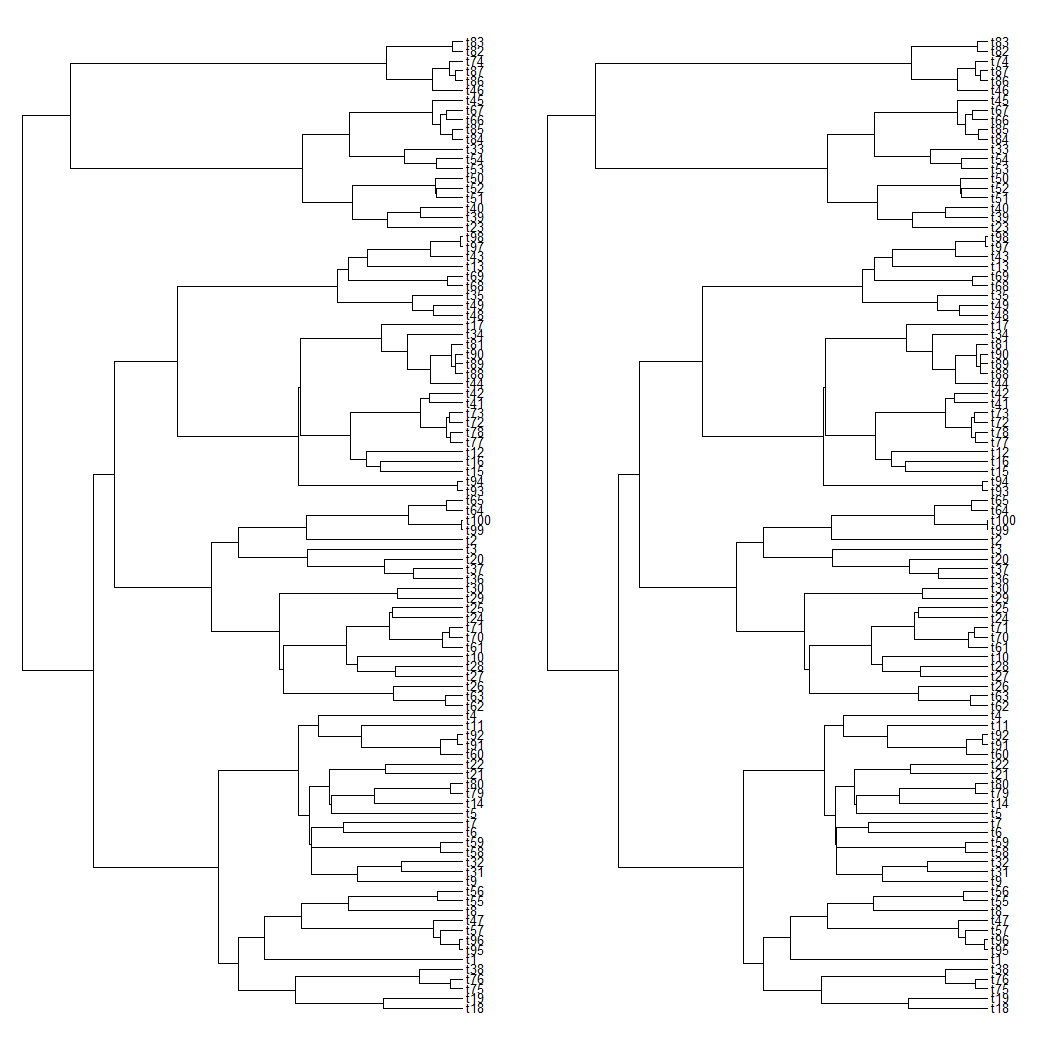## Sunday, June 5, 2022

### Follow-up on the sensitivity of Blomberg's K to terminal edge lengths of the tree

Yesterday I documented in a post on this blog how a seemingly very small change to the edge lengths of our tree could have a very large effect on our measurement of phylogenetic signal using Blomberg et al.'s K statistic.

Because I used a very small tree (ten tips) in the example, a Twitter follower, Gustavo Paterno, asked the following, very natural question: [Does] the issue become less sensitive with increasing sample size?

Unfortunately, the answer is an emphatic NO. Let's see.

Here I'll simulate a tree with 100 taxa, I'll simulate a trait on that tree under Brownian motion, and then, using code that's similar to what I posted yesterday, I'll shorten all the tips of the tree by slightly less than half the distance between the two most closely related sister taxa.

First, here are my trees:

``````library(phytools)
t1<-pbtree(n=100)
## compute patristic distance matrix
D<-as.dist(cophenetic(t1))
## find distance to cut
slice<-min(D)/2-1e-12
t2<-treeSlice(t1,max(nodeHeights(t1))-slice,
orientation="rootwards")
par(mfrow=c(1,2))
plotTree(t1,lwd=1,fsize=0.5)
plotTree(t2,lwd=1,fsize=0.5)
``````Simulate a single character on `tree1`.

``````x<-fastBM(t1)
``````
``````##                t18                t19                t75                t76
##  1.705892864344912  1.866598869750947 -0.197770768667297 -0.692227700756325
##                t38                 t1
## -0.198408749443290 -0.108875374497454
``````

Now compute phylogenetic signal using Blomberg et al.'s K on each of the two trees.

``````phylosig(t1,x,test=TRUE)
``````
``````##
## Phylogenetic signal K : 1.08337
## P-value (based on 1000 randomizations) : 0.001
``````
``````phylosig(t2,x,test=TRUE)
``````
``````##
## Phylogenetic signal K : 0.0000537938
## P-value (based on 1000 randomizations) : 0.014
``````

As before, we see that λ is pretty much unaffected.

``````phylosig(t1,x,method="lambda",test=TRUE)
``````
``````##
## Phylogenetic signal lambda : 1.0005
## logL(lambda) : -123.238
## LR(lambda=0) : 161.014
## P-value (based on LR test) : 6.79424e-37
``````
``````phylosig(t2,x,method="lambda",test=TRUE)
``````
``````##
## Phylogenetic signal lambda : 0.999827
## logL(lambda) : -123.238
## LR(lambda=0) : 161.014
## P-value (based on LR test) : 6.79424e-37
``````

One trick that could help us understand this apparent contradiction a bit better is to see what happens when we transform our second tree by the MLE of λ for that tree, and then compute K on the transformed tree.

Let's check.

``````lambda2<-phylosig(t2,x,method="lambda")\$lambda
phylosig(phytools:::lambdaTree(t2,lambda2),x)
``````
``````##
## Phylogenetic signal K : 1.06701
``````

What? Ha.